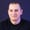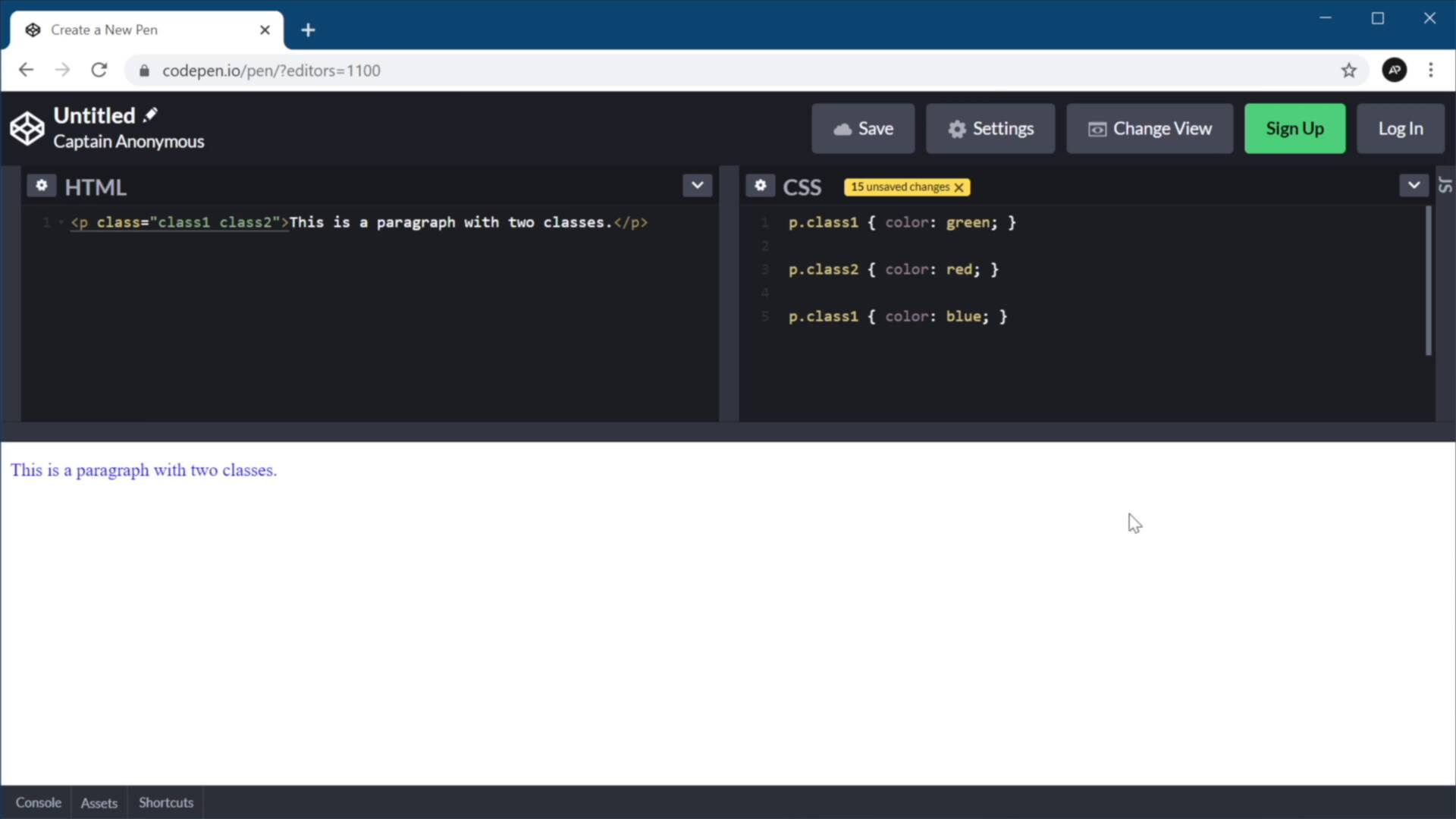FREELessons: 5Length: 27 minutes• Overview
• Transcript

# 2.1 What Is CSS Specificity?

Welcome back to the course. In this lesson, we answer the most obvious question: “What is CSS specificity?”

## 2.Understanding CSS Specificity4 lessons, 25:55

### 2.1 What Is CSS Specificity?

Welcome back to the course. In this lesson, we're gonna answer the most obvious question, what is CSS specificity? To do that, let's go to MDN and see the definition that they give for it. Specificity is the means by which browsers decide which CSS property values are the most relevant to an element and therefore, will be applied. In other words, if you're trying to change the same property using two selectors, then the browser will use specificity to determine which value to use. To understand this better, you need to understand the cascading nature of CSS. As you know CSS stands for Cascading Style Sheets. And cascading means that the order in which rules are applied matters. Let me give you an example. I'm gonna create a quick paragraph here, and I'm gonna add the classes of class 1 and class 2. Now, if I go to my CSS and I say p class 1, color green, the paragraph is gonna be green. If I say p class 2, color red, and that's gonna be red. If I say again, p class 1 color blue, then this paragraph will be blue. So what you need to remember from this is that, if two rules are applied to the same elements, the one that comes last is the one that will be used. So in our case, the paragraph will be blue because this is the last rule being applied to this element. Now this is very easy to understand, but in a real project things are rarely that simple, because you can have different selectors targeting an element, or the same element. So let me show you an example. Here I have a paragraph with an ID and the class. So if I target that paragraph by its ID, let's say it's color blue, and then I target it by its class, you would expect this paragraph to be read because this is the last rule that's targeting that paragraph. But that's not the case here because, targeting that element using an ID selector, has a higher specificity value than targeting that element using a class selector. So the color being applied here is blue, regardless of the order in which these are written in CSS. Now, to understand how specificity works and how it's calculated, we need to understand its hierarchy. So we'll do that in the next lesson, I'll see you there.

Back to the top